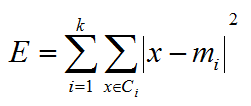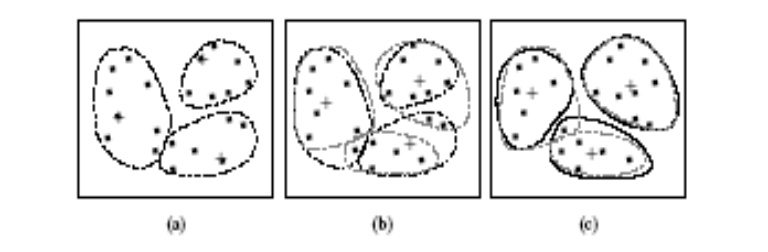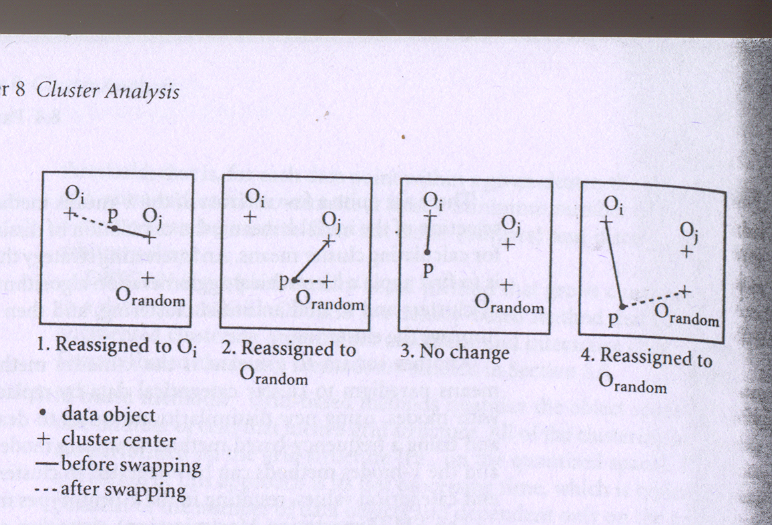# Partitional Clustering - K-Means & K-Medoids

## Partitional Clustering Introduction

(Before reading this you make sure to understand what is clustering)

Given a database of n objects or data tuples, a partitioning method constructs k partitions of the data, where each partition represents a cluster and k <= n.

That is, it classifies the data into k groups, which together satisfy the following requirements
• Each group must contain at least one object,
• Each object must belong to exactly one group.

"Notice that the second requirement can be relaxed in some fuzzy partitioning techniques."

Given k, the number of partitions to construct, a partitioning method creates an initial partition.

It uses iterative relocation technique that attempts to improve the partitioning by moving objects from one group to another.

The general criterion of a good partitioning is that objects in the same cluster are “close” or related to each other, whereas objects of different clusters are “far apart” or very different.

There are various kinds of other criteria for judging the quality of partitions.

To achieve global optimality in partitioning – based clustering it would require the continuous evaluation of all the possible partitions.

1) The k-means algorithm, where each cluster is represented by the mean value of the objects in the cluster.

2) the k-medoids algorithm, where each cluster is represented by one of the objects located near the center of the cluster.

The heuristic clustering methods work well for finding spherical-shaped clusters in small to medium databases.

To find clusters with complex shapes and for clustering very large data sets, partitioning based methods need to be extended.

## Partitioning Algorithms: Basic Concept

Partitioning method: Construct a partition of a database D of n objects into a set of k clusters.

Given a k, find a partition of k clusters that optimizes the chosen partitioning criterion
• Global optimal: Continous evaluation all partitions
• Heuristic methods: k-means and k-medoids algorithms
• k-means (MacQueen’67): Each cluster is represented by the center of the cluster
• k-medoids or PAM (Partition around medoids) (Kaufman & Rousseeuw’87): Each cluster is represented by one of the objects in the cluster

## How Does K-Means Work

First, it randomly selects k of the objects, each of which initially represents a cluster mean or center.

For each of the remaining objects, an object is assigned to the cluster to which it is the most similar, based on the distance between the object and the cluster mean.

It then computes the new mean for each cluster. This process iterates until the criterion function converges.

## K-Means Algorithm

Given k, the k-means algorithm is implemented in 4 steps:
• Partition objects into k nonempty subsets
• Compute seed points as the centroids of the clusters of the current partition.  The centroid is the center (mean point) of the cluster.
• Assign each object to the cluster with the nearest seed point.
• Go back to Step 2, stop when no more new assignment.Here, E is the sum of the square error for all objects in the data set.

x is the point in space representing a given object, and mi is the mean of cluster Ci (both x and mi are multidimensional). In other words, for each object in each cluster, the distance from the object to its cluster center is squared, and the distances are summed.

This criterion tries to make the resulting k clusters as compact and as separate as possible.Suppose that there is a set of objects located in space as depicted in the rectangle.

Let k =3; i.e. the user would like to cluster the object into three clusters.

According to the algorithm, we arbitrarily choose three objects as the three initial cluster centers, were cluster centers are marked by a “+”.

Each object is distributed to a cluster based on the cluster center to which it is the nearest.

Such distribution forms circled by dotted curves.

Relatively efficient: O(tkn), where n is # objects, k is # clusters, and t  is # iterations. Normally, k, t << n.

Each object is distributed to a cluster based on the cluster center to which it is the nearest.

Applicable only when mean is defined, then what about categorical data?

Need to specify k, the number of clusters, in advance.

Unable to handle noisy data and outliers.

Not suitable to discover clusters with non-convex shapes.

## K-Medoids Clustering

A medoid can be defined as that object of a cluster, whose average dissimilarity to all the objects in the cluster is minimal i.e. it is a most centrally located point in the given dataset.

K-Medoids: Instead of taking the mean value of the object in a cluster as a reference point, medoids can be used, which is the most centrally located object in a cluster.

The basic strategy of k-medoids clustering algorithms is to find k clusters in n objects by first arbitrarily finding a representative object (the medoid) for each cluster.

Each remaining object is clustered with the medoid to which it is most similar.

The strategy then iteratively replaces one of the medoids by one of the non-medoids as long as the quality of the resulting clustering is improved.

This quality is estimated by using a cost function that measures the average dissimilarity between an object and the medoid of its cluster.

To determine whether a non-medoid object is "Oi" random is a good replacement for a current medoid "Oj", the following four cases are examined for each of the non-medoid objects "P".Case 1: "P" currently belongs to medoid "Oj", If "Oj" is replaced by "Orandom", as a medoid and "P" is closest to one of "Oi", it do not belong "j", then "P" is assigned to "Oi".

Case 2: "P" currently belongs to medoid "Oj". If "Oj" is replaced by "Orandom" as medoid and "P" is closest to "Orandom", then "P" is reassigned to "Orandom".

Case 3: "P" currently belongs to medoid "Oi", it does not belong "j". If "Oj" is replaced by "Orandom" as a medoid and "P" is still closest to "Oi", then the assignment does not change.

Case 4: "P" currently belongs to medoid "Oi", it does not belong to "j". If "Oj" is replaced by "Orandom" as a medoid and "P" is closest to "Orandom", then "P" is reassigned to "Orandom".

## Which Is More Robust -- K-Means or K-Medoids

The k-medoids method is more robust than k-means in the presence of noise and outliers because a medoid is less influenced by outliers or other extreme values than a mean.

However, its processing is more costly than the k-means method. Both methods require the user to specify k, the number of clusters.

Aside from using the mean or the medoid as a measure of cluster center, other alternative measures are also commonly used in partitioning clustering methods.

The median can be used, resulting in the k-median method, where the median or “middle value” is taken for each ordered attribute. Alternatively, in the k-modes method, the most frequent value for each attribute is used.

## Summary

Partitional Clustering -> Given a database of n objects or data tuples, a partitioning method constructs k partitions of the data, where each partition represents a cluster and k <= n.

Subscribe us for more content on Data.